### Home > CALC > Chapter 8 > Lesson 8.3.1 > Problem8-101

8-101.
1.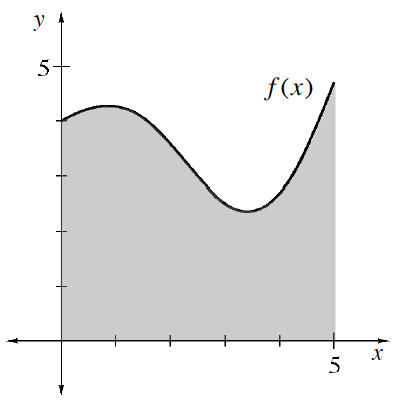The graph at right is f(x) =x cos x + 4 for 0 ≤ x ≤ 5. Write an integral that could compute the volume of the solid when this region is rotated about: Homework Help ✎

1. The x-axis.

2. The y-axis.

3. The line x = 6.

4. The line y = −7.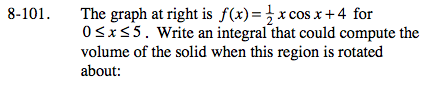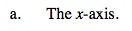Vertical rectangles.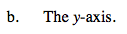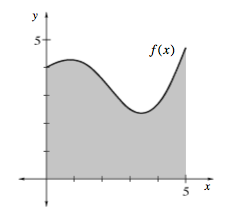Horizontal rectangles would be very difficult (because the inverse of f(x) is NOT a function). So use the Shell Method.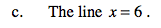By shells:

Where did the (6 − x) come from?
Recall that each cylindrical shell is a prism with dimensions: (base)(height)(width) = (2πr)(f(x))(dx).
Well, (6 − x) represents the r (radius) in the circumference part of the formula: C = 2πr.
If we were rotating about the y-axis (as in part (b)), then the radius would be the same as the bounds, x.
But since we are rotating about a line that is parallel to the y-axis, we do not want the radius to be the same as the bounds.
When we plug in the lower bound (x = 0), we actually want a radius that is 6.
And when we plug in the upper bound (x = 5), we actually want a radius that is 1.
(6 − x) will make this work!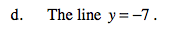Vertical rectangles with a hole in the center.